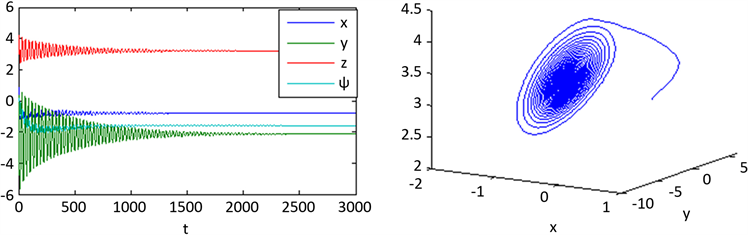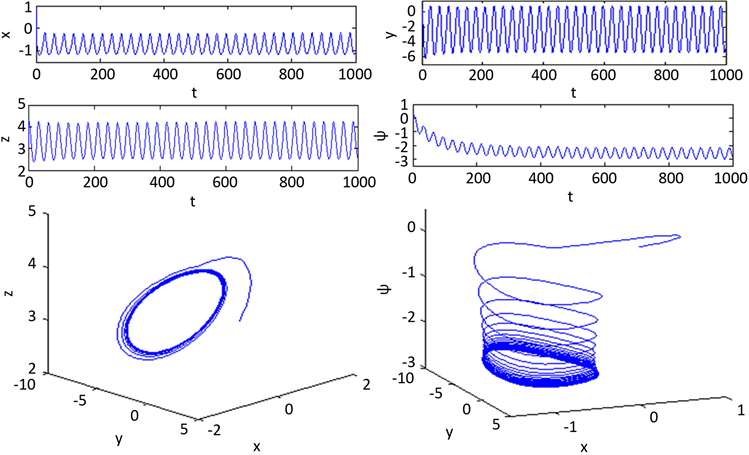# 具有时滞的eHR神经元模型稳定性及Hopf分岔分析Stability and Hopf Bifurcation Analysis of eHR Neuron Model with Time-Delay

DOI: 10.12677/AAM.2018.712189, PDF, HTML, XML, 下载: 442  浏览: 591

Abstract: In order to study the complex dynamic behavior of time-delayed neuron system, the time-delay term is introduced on the basis of eHR neuron system. By analyzing the characteristic equation of the linearized eHR model system at the unique equilibrium point, a critical value is obtained, so that Hopf bifurcation occurs when the value exceeds it, and the system is asymptotically stable when the value is less than it. In addition, the stability and bifurcation direction of the bifurcation periodic solution are given by the central manifold theorem and other theories. Finally, some nu-merical simulations are given to verify the conclusions.

1. 引言

$\left\{\begin{array}{l}\stackrel{˙}{x}=y-a{x}^{3}+b{x}^{2}-z+{I}_{ext}\\ \stackrel{˙}{y}=c-d{x}^{2}-y\\ \stackrel{˙}{z}=r\left[s\left(x-{x}_{0}\right)-z\right]\end{array}$ (1)

$\left\{\begin{array}{l}\stackrel{˙}{x}=y-a{x}^{3}+b{x}^{2}-z+{I}_{ext}\\ \stackrel{˙}{y}=c-d{x}^{2}-y-ew\\ \stackrel{˙}{z}=r\left[s\left(x-{x}_{0}\right)-z\right]\\ \stackrel{˙}{w}=h\left[f\left(y+g\right)-pw\right]\end{array}$ (2)

2. 模型介绍

$\left\{\begin{array}{l}\stackrel{˙}{x}=y\left(t-\tau \right)-a{x}^{3}+b{x}^{2}-z+{I}_{ext}\\ \stackrel{˙}{y}=c-d{x}^{2}-y\left(t-\tau \right)-ew\\ \stackrel{˙}{z}=r\left[s\left(x-{x}_{0}\right)-z\right]\\ \stackrel{˙}{w}=h\left[f\left(y\left(t-\tau \right)+g\right)-pw\right]\end{array}$ (3)

3. 平衡点分析和Hopf分岔存在性稳定性分析

$\left\{\begin{array}{l}\stackrel{˙}{x}=y\left(t-\tau \right)-a{x}^{3}+\left(b-3c{x}^{*}\right){x}^{2}+\left(2b{x}^{*}-3c{x}^{*2}\right)x-z\\ \stackrel{˙}{y}=-d{x}^{2}-2d{x}^{*}x-y\left(t-\tau \right)-ew\\ \stackrel{˙}{z}=rsx-rz\\ \stackrel{˙}{w}=hfy\left(t-\tau \right)-hpw\end{array}$ (4)

$\left\{\begin{array}{l}\stackrel{˙}{x}=y\left(t-\tau \right)+\left(2b{x}^{*}-3a{x}^{*2}\right)x-z\\ \stackrel{˙}{y}=-2d{x}^{*}x-y\left(t-\tau \right)-ew\\ \stackrel{˙}{z}=rsx\left(t-{\tau }_{1}\right)-rz\\ \stackrel{˙}{w}=hfy\left(t-{\tau }_{2}\right)-hpw\end{array}$ (5)

$A=\left(\begin{array}{cccc}2b{x}^{*}-3a{x}^{*2}& {e}^{-\lambda \tau }& -1& 0\\ -2d{x}^{*}& -{e}^{-\lambda \tau }& 0& -e\\ rs& 0& -r& 0\\ 0& hf{e}^{-\lambda \tau }& 0& -hp\end{array}\right)$

${\lambda }^{4}+{k}_{3}{\lambda }^{3}+{k}_{2}{\lambda }^{2}+{k}_{1}\lambda +\left({\lambda }^{3}+{n}_{2}{\lambda }^{2}+{n}_{1}\lambda +{n}_{0}\right){e}^{-\lambda \tau }=0$ (6)

$\begin{array}{l}{k}_{3}=3a{x}^{2}-2bx+hp+r,\text{\hspace{0.17em}}\text{\hspace{0.17em}}{k}_{2}=3\left(ahp+ar\right){x}^{2}-2\left(bhpx+br\right)x+hpr+rs,\\ {k}_{1}=3ahpr{x}^{2}-2bhprx+hprs,\text{\hspace{0.17em}}\text{\hspace{0.17em}}{n}_{2}=3a{x}^{2}+2\left(d-b\right)x+hfe+hp+r,\\ {n}_{1}=3\left(aefh+ahp+ar\right){x}^{2}-2\left(befh+bhp-dhp+br-dr\right)x+efhr+hpr+rs,\\ {n}_{0}=3\left(aefhr+ahpr\right){x}^{2}-2\left(befhr-dhpr\right)x-2bhpr+efhrs+hprs\end{array}$

${\lambda }^{4}+{q}_{13}{\lambda }^{3}+{q}_{12}{\lambda }^{2}+{q}_{11}\lambda +{q}_{10}=0$ (7)

${q}_{13}={k}_{3}+1,\text{\hspace{0.17em}}{q}_{12}={k}_{2}+{n}_{2},\text{\hspace{0.17em}}{q}_{11}={k}_{1}+{n}_{1},\text{\hspace{0.17em}}{q}_{10}={n}_{0}$

(H1) ${q}_{13}>0,{q}_{12}{q}_{13}>{q}_{11},{q}_{11}{q}_{12}{q}_{13}>{q}_{10}{q}_{13}^{2}+{q}_{11}^{2}$

$\lambda =i\omega$ 是方程(6)的根，则有

${\omega }^{4}-i{k}_{3}{\omega }^{3}-{k}_{2}{\omega }^{2}+i{k}_{1}\omega +\left(-i{\omega }^{3}-{n}_{2}{\omega }^{2}+i{n}_{1}\omega +{n}_{0}\right)\left[\mathrm{cos}\left(\omega \tau \right)-i\mathrm{sin}\left(\omega \tau \right)\right]=0$ (8)

$\left\{\begin{array}{l}\left({n}_{0}-{n}_{2}{\omega }^{2}\right)\mathrm{cos}\left(\omega \tau \right)+\left({n}_{1}\omega -{\omega }^{3}\right)\mathrm{sin}\left(\omega \tau \right)={k}_{2}{\omega }^{2}-{\omega }^{4}\\ \left({n}_{1}\omega -{\omega }^{3}\right)\mathrm{cos}\left(\omega \tau \right)+\left({n}_{2}{\omega }^{2}-{n}_{0}\right)\mathrm{sin}\left(\omega \tau \right)={k}_{3}{\omega }^{3}-{k}_{1}\omega \end{array}$

$\left\{\begin{array}{l}\mathrm{sin}\left(\omega {\tau }_{1}\right)=\frac{{\omega }^{7}+{A}_{2}{\omega }^{5}+{A}_{1}{\omega }^{3}+{A}_{0}\omega }{{\omega }^{6}+{B}_{2}{\omega }^{4}+{B}_{1}{\omega }^{2}+{B}_{0}}\\ \mathrm{cos}\left(\omega {\tau }_{1}\right)=\frac{{A}_{5}{\omega }^{6}+{A}_{4}{\omega }^{4}+{A}_{3}{\omega }^{2}}{{\omega }^{6}+{B}_{2}{\omega }^{4}+{B}_{1}{\omega }^{2}+{B}_{0}}\end{array}$ (9)

$\begin{array}{l}{A}_{0}={n}_{0}{k}_{1},{A}_{1}={n}_{1}{k}_{2}-{n}_{0}{k}_{3}-{n}_{2}{k}_{1},{A}_{2}={n}_{2}{k}_{3}-{n}_{1}-{k}_{2},\\ {A}_{3}={n}_{0}{k}_{2}-{n}_{1}{k}_{2},{A}_{4}={n}_{1}{k}_{3}-{n}_{2}{k}_{2}+{k}_{1}-{n}_{0},{A}_{5}={n}_{2}-{k}_{3},\\ {B}_{0}={n}_{0}^{2},{B}_{1}={n}_{1}^{2}-2{n}_{0}{n}_{2},{B}_{2}={n}_{2}^{2}-2{n}_{1}\end{array}$

${\omega }^{8}+{b}_{3}{\omega }^{6}+{b}_{2}{\omega }^{4}+{b}_{1}{\omega }^{2}+{b}_{0}=0$ (10)

$\begin{array}{l}{b}_{3}={k}_{3}^{2}-2{k}_{2}-1,\text{\hspace{0.17em}}\text{\hspace{0.17em}}{b}_{2}={k}_{2}^{2}-2{k}_{1}{k}_{3}-{n}_{2}^{2}+2{n}_{1},\\ {b}_{1}={k}_{1}^{2}+2{n}_{0}{n}_{2}-{n}_{1}^{2},\text{\hspace{0.17em}}\text{\hspace{0.17em}}{b}_{0}=-{n}_{0}^{2}\end{array}$

$\eta ={\omega }^{2}$ ，则方程(10)变为：

${\eta }^{4}+{b}_{3}{\eta }^{3}+{b}_{2}{\eta }^{2}+{b}_{1}\eta +{b}_{0}=0$ (11)

$f\left(\eta \right)={\eta }^{4}+{b}_{3}{\eta }^{3}+{b}_{2}{\eta }^{2}+{b}_{1}\eta +{b}_{0}$

${\tau }_{2k}^{\left(i\right)}=\frac{1}{{\omega }_{k}}\left\{\mathrm{arccos}\left(\frac{{A}_{25}{\omega }^{6}+{A}_{24}{\omega }^{4}+{A}_{23}{\omega }^{2}}{{\omega }^{6}+{B}_{22}{\omega }^{4}+{B}_{21}{\omega }^{2}+{B}_{20}}\right)+2i\text{π}\right\},k=1,2,3,4;i=0,1,2,\cdots$

$\lambda \left(\tau \right)=\alpha \left(\tau \right)+i\omega \left(\tau \right)$ 是特征方程(6)在时滞 $\tau ={\tau }_{20}$ 附近满足 $\alpha \left({\tau }_{20}\right)=0$$\omega \left({\tau }_{20}\right)={\omega }_{20}$ 的根，可以得到如下横截性条件。

${\eta }_{k}={\omega }_{k}^{2}$${h}^{\prime }\left({z}_{k}\right)\ne 0$ ，则 ${\left(\frac{\text{d}\left(\mathrm{Re}\lambda \right)}{\text{d}\tau }\right)}_{\tau ={\tau }_{2k}^{\left(i\right)}}\ne 0$${\left(\frac{\text{d}\left(\mathrm{Re}\lambda \right)}{\text{d}\tau }\right)}_{\tau ={\tau }_{2k}^{\left(i\right)}}$${f}^{\prime }\left({\eta }_{k}\right)$ 有相同的符号。

ii) 若 ${f}^{\prime }\left({\eta }_{k}\right)\ne 0$ ，在临界值 ${\tau }_{2}={\tau }_{2k}^{\left(i\right)}$ 时，系统(3)在平衡点 ${E}^{*}=\left({x}^{*},{y}^{*},{z}^{*},{w}^{*}\right)$ 处发生Hopf分岔，即一组非常数周期解会从平衡点分岔出来。

4. Hopf分岔的方向及稳定性

$\stackrel{˙}{x}\left(t\right)={L}_{\mu }\left({x}_{t}\right)+F\left(\mu ,{x}_{t}\right)$

${L}_{\mu }\left(\varphi \right)=\left({\tau }_{0}+\mu \right)B\left(\begin{array}{c}{\varphi }_{1}\left(0\right)\\ {\varphi }_{2}\left(0\right)\\ {\varphi }_{3}\left(0\right)\\ {\varphi }_{4}\left(0\right)\end{array}\right)+\left({\tau }_{0}+\mu \right)C\left(\begin{array}{c}{\varphi }_{1}\left(-\frac{{\tau }_{2*}}{{\tau }_{0}}\right)\\ {\varphi }_{2}\left(-\frac{{\tau }_{2*}}{{\tau }_{0}}\right)\\ {\varphi }_{3}\left(-\frac{{\tau }_{2*}}{{\tau }_{0}}\right)\\ {\varphi }_{4}\left(-\frac{{\tau }_{2*}}{{\tau }_{0}}\right)\end{array}\right)+\left({\tau }_{0}+\mu \right)D\left(\begin{array}{c}{\varphi }_{1}\left(-1\right)\\ {\varphi }_{2}\left(-1\right)\\ {\varphi }_{3}\left(-1\right)\\ {\varphi }_{4}\left(-1\right)\end{array}\right)$

$F\left(\mu ,\varphi \right)=\left({\tau }_{0}+\mu \right)\left(\begin{array}{c}\left(b-3a{x}^{*}\right){\varphi }_{1}^{2}\left(0\right)-a{\varphi }_{1}^{3}\left(0\right)\\ -d{\varphi }_{1}^{2}\left(0\right)\\ 0\\ 0\end{array}\right)$

$B=\left(\begin{array}{cccc}2bx-3a{x}^{2}& 0& -d& 0\\ -2dx& 0& 0& -e\\ 0& 0& -r& 0\\ 0& 0& 0& -hp\end{array}\right),C=\left(\begin{array}{cccc}0& 0& 0& 0\\ 0& -1& 0& 0\\ 0& 0& 0& 0\\ 0& 0& 0& 0\end{array}\right),D=\left(\begin{array}{cccc}0& 1& 0& 0\\ 0& 0& 0& 0\\ rs& 0& 0& 0\\ 0& hf& 0& 0\end{array}\right)$

${L}_{\mu }\varphi ={\int }_{-1}^{0}\text{d}\eta \left(\theta ,\mu \right)\varphi \left(\theta \right)\text{\hspace{0.17em}},\text{\hspace{0.17em}}\varphi \in C\left(\left[-1,0\right],{R}^{4}\right)$

$\eta \left(\theta ,\mu \right)=\left({\tau }_{0}+\mu \right)\left(\begin{array}{cccc}2bx-3a{x}^{2}& 0& -d& 0\\ -2dx& 0& 0& -e\\ 0& 0& -r& 0\\ 0& 0& 0& -hp\end{array}\right)\delta \left(\theta \right)-\left({\tau }_{0}+\mu \right)\left(\begin{array}{cccc}0& 1& 0& 0\\ 0& -1& 0& 0\\ rs& 0& 0& 0\\ 0& hf& 0& 0\end{array}\right)\delta \left(\theta +1\right)$

$A\left(\mu \right)\varphi \left(\theta \right)=\left\{\begin{array}{ll}\frac{\text{d}\varphi \left(\theta \right)}{\text{d}\theta }，\hfill & \theta \in \left[-1,0\right)\hfill \\ {\int }_{-1}^{0}\text{d}\eta \left(\theta ,\mu \right)\varphi \left(\theta \right)，\hfill & \theta =0\hfill \end{array}$

$R\left(\mu \right)\varphi \left(\theta \right)=\left\{\begin{array}{ll}0,\hfill & \theta \in \left[-1,0\right)\hfill \\ F\left(\mu ,\varphi \right)，\hfill & \theta =0\hfill \end{array}$

${\stackrel{˙}{x}}_{t}=A\left(\mu \right){x}_{t}+R\left(\mu \right){x}_{t}$

${A}^{*}\left(\mu \right)\psi \left(s\right)=\left\{\begin{array}{ll}-\frac{\text{d}\psi \left(s\right)}{\text{d}s},\hfill & s\in \left(0,1\right]\hfill \\ {\int }_{-1}^{0}\text{d}\eta \left(t,0\right)\psi \left(-t\right)，\hfill & s=0\hfill \end{array}$

$〈\psi \left(s\right),\varphi \left(\theta \right)〉=\stackrel{¯}{\psi }\left(0\right)\varphi \left(0\right)-{\int }_{-1}^{0}{\int }_{\xi =0}^{\theta }{\stackrel{¯}{\psi }}^{\text{T}}\left(\xi -\theta \right)\text{d}\eta \left(\theta \right)\varphi \left(\xi \right)\text{d}\xi$

${g}_{20}={g}_{11}={g}_{02}=2\stackrel{¯}{P}{\tau }_{{2}_{0}}\left(b-3a{x}^{*}-d\stackrel{¯}{{v}_{1}^{*}}\right)$

${g}_{21}=2\stackrel{¯}{P}{\tau }_{{2}_{0}}\left[\left(b-3a{x}^{*}\right)\left({W}_{20}^{\left(1\right)}+2{W}_{11}^{\left(1\right)}\right)-3a-f\left({W}_{20}^{\left(1\right)}+2{W}_{11}^{\left(1\right)}\right)\stackrel{¯}{{v}_{1}^{*}}\right]$

${W}_{20}\left(\theta \right)=\frac{i{g}_{20}}{{\omega }_{0}{\tau }_{0}}q\left(0\right){e}^{i{\omega }_{0}{\tau }_{0}\theta }+\frac{i{\stackrel{¯}{g}}_{02}}{3{\omega }_{0}{\tau }_{0}}\stackrel{¯}{q}\left(0\right){e}^{-i{\omega }_{0}{\tau }_{0}\theta }+{E}_{1}{e}^{2i{\omega }_{0}{\tau }_{0}\theta }$

${W}_{11}\left(\theta \right)=-\frac{i{g}_{11}}{{\omega }_{0}{\tau }_{0}}q\left(0\right){e}^{i{\omega }_{0}{\tau }_{0}\theta }+\frac{i{\stackrel{¯}{g}}_{11}}{{\omega }_{0}{\tau }_{0}}\stackrel{¯}{q}\left(0\right){e}^{-i{\omega }_{0}{\tau }_{0}\theta }+{E}_{2}$

${E}_{1}={\left(\begin{array}{cccc}2i{\omega }_{0}{\tau }_{20}-\left(2b{x}^{*}-3a{x}^{*2}\right)& -{e}^{-2i{\omega }_{0}{\tau }_{2}^{*}}& d& 0\\ 2d{x}^{*}& 2i{\omega }_{0}{\tau }_{20}+{e}^{-2i{\omega }_{0}{\tau }_{2}^{*}}& 0& e\\ -rs{e}^{-2i{\omega }_{0}{\tau }_{20}}& 0& 2i{\omega }_{0}{\tau }_{20}+r& 0\\ 0& -hf{e}^{-2i{\omega }_{0}{\tau }_{2}^{*}}& 0& 2i{\omega }_{0}{\tau }_{20}+hp\end{array}\right)}^{-1}\left(\begin{array}{c}2\left(b-3a{x}^{*}\right)\\ -2d\stackrel{¯}{{v}_{1}^{*}}\\ 0\\ 0\end{array}\right)$

${E}_{2}=-{\left(\begin{array}{cccc}2b{x}^{*}-3a{x}^{*2}& a& -d& 0\\ -2d{x}^{*}& -1& 0& -e\\ rs& 0& -r& 0\\ 0& hp& 0& -hp\end{array}\right)}^{-1}\left(\begin{array}{c}2\left(b-3a{x}^{*}\right)\\ -2d\stackrel{¯}{{v}_{1}^{*}}\\ 0\\ 0\end{array}\right)$

$\left\{\begin{array}{l}{c}_{1}\left(0\right)=\frac{i}{{\omega }_{0}{\tau }_{0}}\left({g}_{11}{g}_{20}-2{|{g}_{11}|}^{2}-\frac{{|{g}_{02}|}^{2}}{3}\right)+\frac{{g}_{21}}{2}\\ {\mu }_{2}=-\frac{\mathrm{Re}\left({c}_{1}\left(0\right)\right)}{\mathrm{Re}\left({{\lambda }^{\prime }}_{0}\left({\tau }_{0}\right)\right)}\\ {\beta }_{2}=2\mathrm{Re}\left({c}_{1}\left(0\right)\right)\\ {T}_{2}=-\frac{\mathrm{Im}\left({c}_{1}\left(0\right)\right)+{\mu }_{2}\mathrm{Im}\left({{\lambda }^{\prime }}_{0}\left({\tau }_{0}\right)\right)}{{\omega }_{0}{\tau }_{0}}\end{array}$

$\tau >{\tau }_{{2}_{0}}\left(\tau <{\tau }_{{2}_{0}}\right)$ 时，分岔周期解存在(不存在)；若 ${T}_{2}>0\left({T}_{2}<0\right)$ ，周期解的周期增加(减小)； ${\beta }_{2}>0\left({\beta }_{2}<0\right)$ ，在此中心流形上，周期解是渐进稳定的(不稳定的)。

5. 数值模拟

$\left\{\begin{array}{l}\stackrel{˙}{x}=y\left(t-\tau \right)-{x}^{3}+3{x}^{2}-z+2.924976\\ \stackrel{˙}{y}=1.01-5.0128{x}^{2}-y\left(t-\tau \right)-0.0278w\\ \stackrel{˙}{z}=0.12\left[3.966\left(x-1.605\right)-z\right]\\ \stackrel{˙}{w}=0.009\left[3\left(y\left(t-\tau \right)+1.619\right)-0.9573w\right]\end{array}$ (2)

$\begin{array}{l}{q}_{13}=1.949051>0,{q}_{12}{q}_{13}=13.211406>{q}_{11}=1.231740,\\ {q}_{11}{q}_{12}{q}_{13}=16.273022>{q}_{10}{q}_{13}^{2}+{q}_{11}^{2}=1.553386\end{array}$

$\tau \ne 0$ 时，由情况2的理论方法计算得 ${\tau }_{{2}_{0}}=0.140347$ ，由引理1可得满足横截性条件。当 $\tau =0.18>{\tau }_{{2}_{0}}$ 时，系统是不稳定的，当 $\tau$ 穿过每一个临界值 ${\tau }_{{2}_{j}}\left(j=0,1,2,\cdots \right)$ 是，系统(3)的一个稳定周期Figure 1. τ = 0, The time serise and phase diagrams of x(t), y(t), z(t), w(t) at the initial values (0.3, 0.3, 3.0, 0.05) show that the equilibrium point P (0.881210, 2.781651, 2.870551, 3.643691) are asymptotically stableFigure 2. $\tau =0.18>{\tau }_{{2}_{0}}$ ,The time serise and phase diagrams of $x\left(t\right),y\left(t\right),z\left(t\right),w\left(t\right)$ at the initial values (0.3, 0.3, 3.1, 0.1) show that The unique equilibrium point P (0.881210, 2.781651, 2.870551, 3.643691) is unstable and a stable periodic solution bifurcates from P

6. 结束语Figure 3. $\tau =0.007<{\tau }_{{2}_{0}}$ , The time serise and phase diagrams of $x\left(t\right),y\left(t\right),z\left(t\right),w\left(t\right)$ at the initial values (0.3, 0.3, 6.1, 0.1) show that the equilibrium point P (0.881210, 2.781651, 2.870551, 3.643691) are asymptotically stable

  Wang, H.X., Wang, Q.Y. and Zhang, Y.H. (2014) Bifurcation Analysis for Hindmarsh-Rose Neuronal Model with Time-Delayed Feedback Control and Application to Chaos Control. Technological Sciences, 5, 872-878.  Cheng, Z.S., Xie, K.H., et al. (2018) Stability and Hopf Bifurcation of Three-Triangle Neural Networks with Delays. Neuro-computing, 322, 206-215. https://doi.org/10.1016/j.neucom.2018.09.063  曹淑红, 段利霞, 唐旭晖, 赵勇. 具有时滞的藕合Hindmarsh-Rose神经元系统的放电模式[J]. 力学与控制学报, 2012, 10(1): 88-91.  Hodgkin, A.L. and Huxley, A.F. (1952) A Quantitative Description of Membrane Current and Its Application to Conduction and Excitation in Nerve. The Journal of Physiology, 117, 500-544. https://doi.org/10.1113/jphysiol.1952.sp004764  Hindmarsh, J.L. and Rose, R.M. (1984) A Model of Neu-ronal Bursting Using Three Coupled First-Order Differential Equations. Proceedings of the Royal Society of London, 221, 87-102. https://doi.org/10.1098/rspb.1984.0024  Song, X.L., Wang, C.N., Ma, J., et al. (2015) Transition of Electric Activity of Neurons Induced by Chemical and Electric Autapses. Science China Technological Sciences, 58, 1007-1014. https://doi.org/10.1007/s11431-015-5826-z  Yang, Z.Q. and Hao, L.J. (2014) Dynamics of Dif-ferent Compound Bursting in Two Phantom Bursting Mechanism Models. Science China Technological Sciences, 57, 885-892. https://doi.org/10.1007/s11431-014-5543-z  Lv, M., Wang, C., Ren, G., et al. (2016) Model of Electrical Activity in a Neuron under Magnetic Flow Effect. Nonlinear Dynamics, 85, 1479-1490. https://doi.org/10.1007/s11071-016-2773-6  Xie, Y., Kang, Y.M., Liu, Y., et al. (2014) Firing Properties and Synchronization Rate in Fractional-Order Hindmarsh-Rose Model Neurons. Science China Technological Sciences, 57, 914-922.  Shi, M. and Wang, Z.H. (2014) Abundant Bursting Patterns of a Fractional-Order Morris-Lecar Neuron Model. Communications in Nonlinear Science and Numerical Simulation, 19, 1956-1969. https://doi.org/10.1016/j.cnsns.2013.10.032  邬开俊. Hindmarsh-Rose神经元模型的双参数分岔特性及耦合同步研究[D]: [博士学位论文]. 兰州: 兰州交通大学, 2017.